# 14 W Incandescent Bulb

 We are testing a 14 Watt Incandescent Bulb at  220 Volts Mains AC input. We are using Embuilt  SPEA-1 to test the Bulb properties.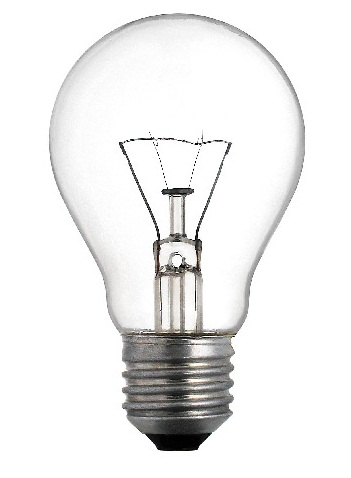Specifications : 230 Volts, 14 Watt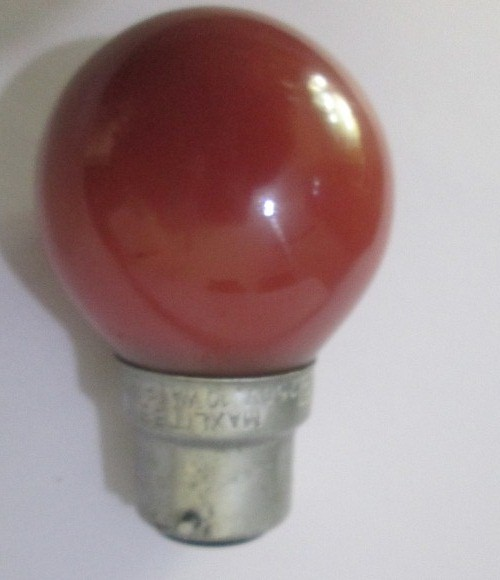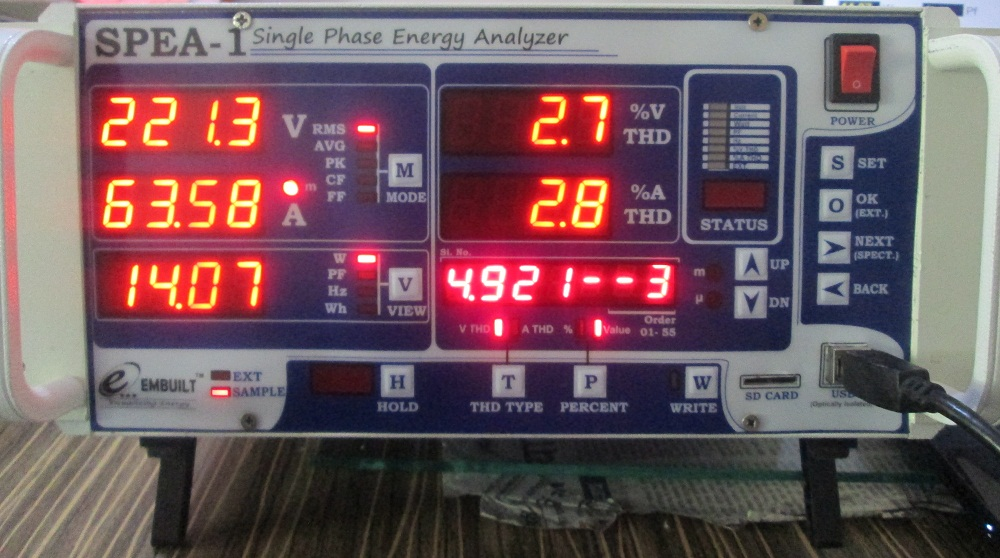When power is on the following results are found –

 Vrms- 221.3 Volts VTHD% -2.7% Arms -63.58mA ATHD% -2.8% Watt -14.07Watt V3rd Harmonics Value – 4.921 Volts

Here we can see that VTHD is 2.7% which means Input Supply has 2.7% distortion in Voltage.

ATHD % is 2.8%. Here question is if bulb is resistive so why there is Amps THD.

Answer is ATHD is sum of VTHD and Distortion caused by load. So NET THD is 0.1%.

3rd harmonics of Voltage is 6.329 Volts.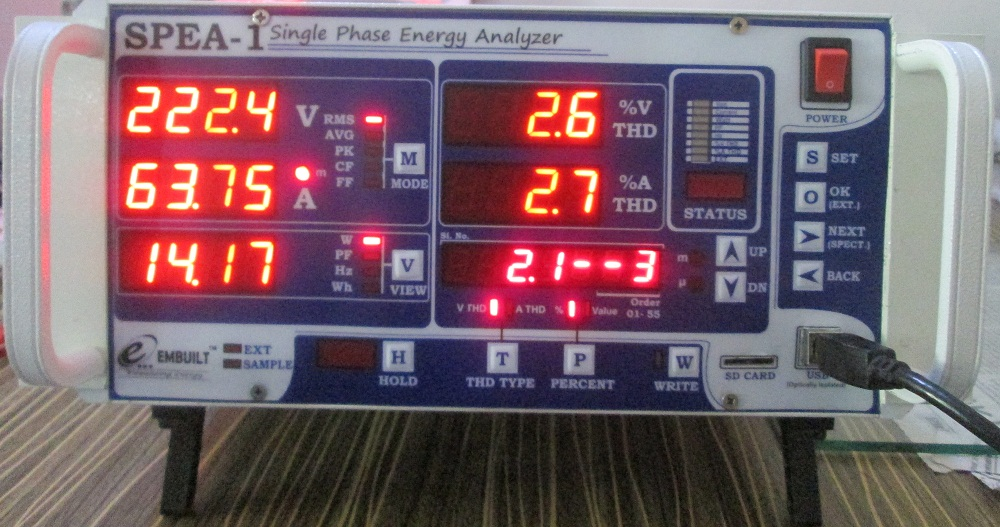Here we can see the 3rd harmnonics of voltage in % of first harmonics. 3rd Voltage harmonics is 2.1% of Vrms Harmonics.

Here we note that ideally first harmonics is to be equal of Vrms and other order harmonics are to 0.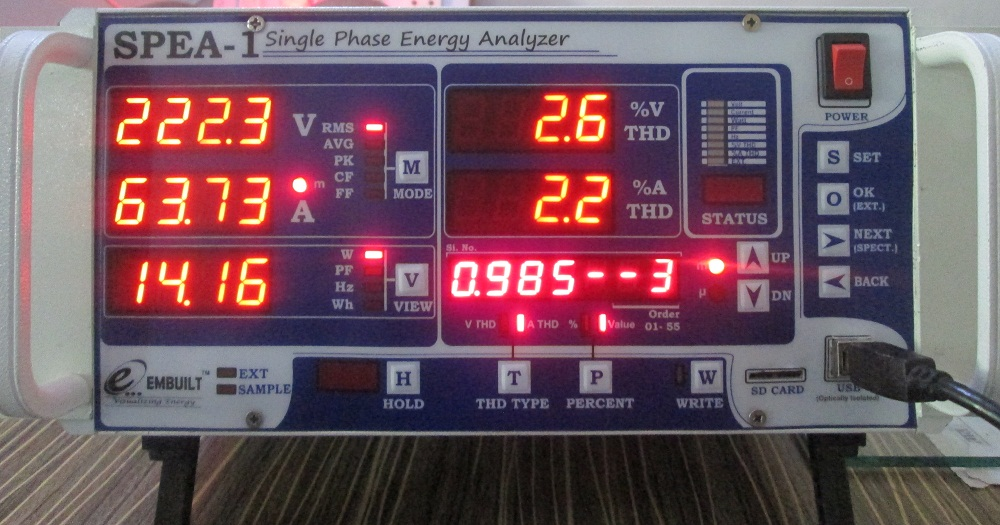Here we can see the 3rd harmonics Value of Amps. It is 0.985mA.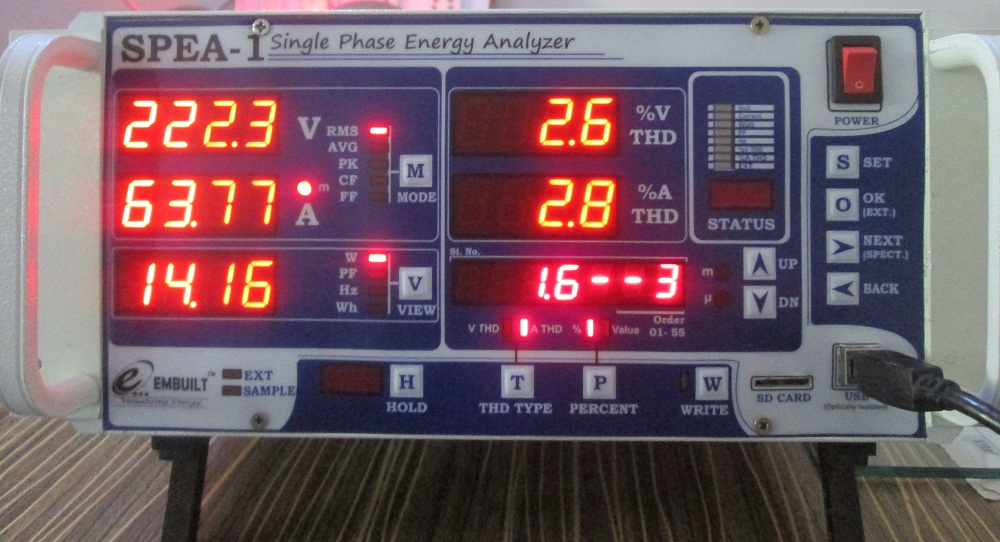In the above image 3rd Harmonics of Amps shows in display 6. It is 1.6% of Arms (63.77mA).

Other parameters can also seen on SPEA-1.

You can also view readings on the SPEA-1 Software.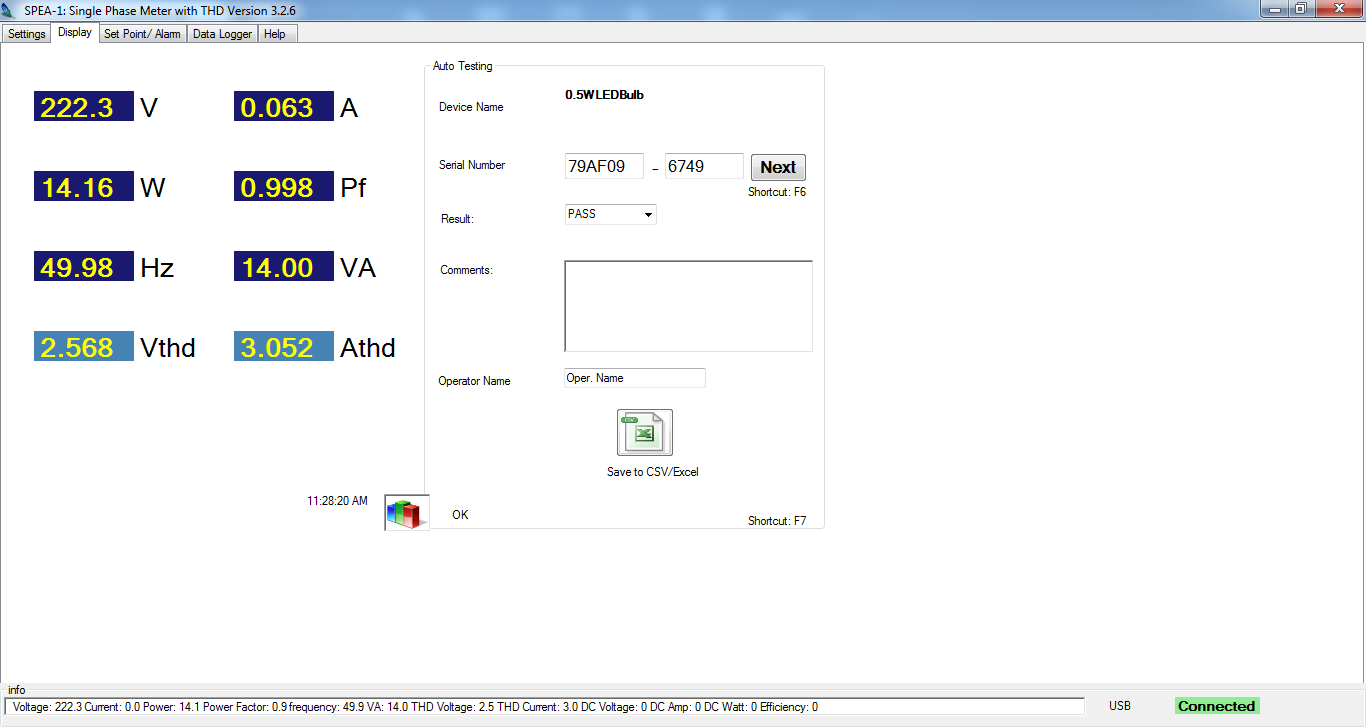Softare is connected to SPEA-1 for real time data viewing and reporting.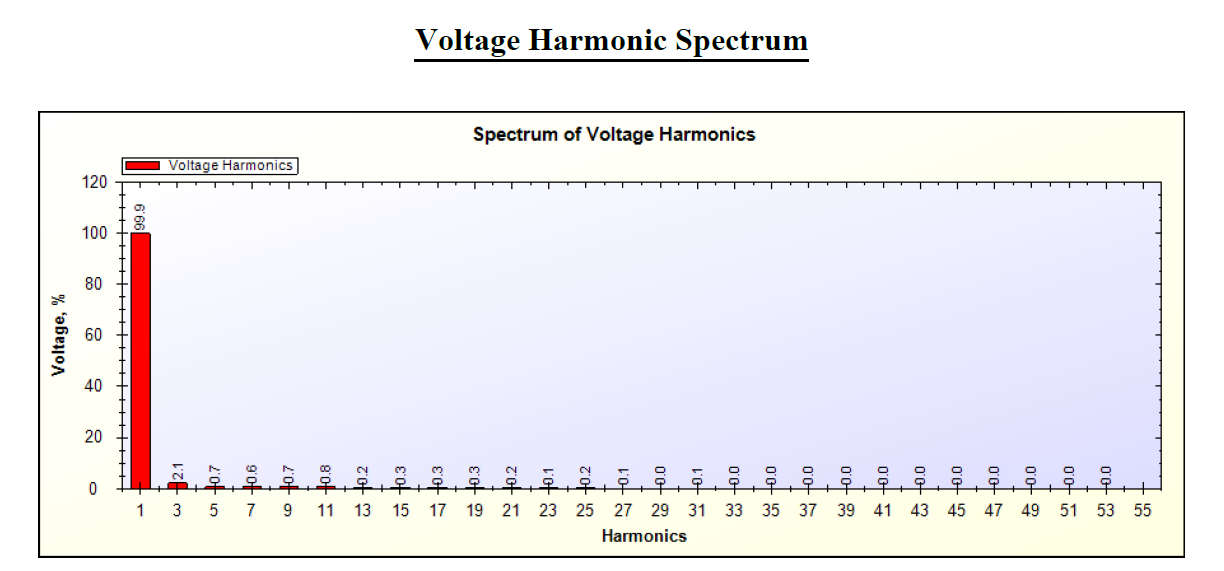Above is the voltage harmonics spectrum. All values are given in % of Vrms.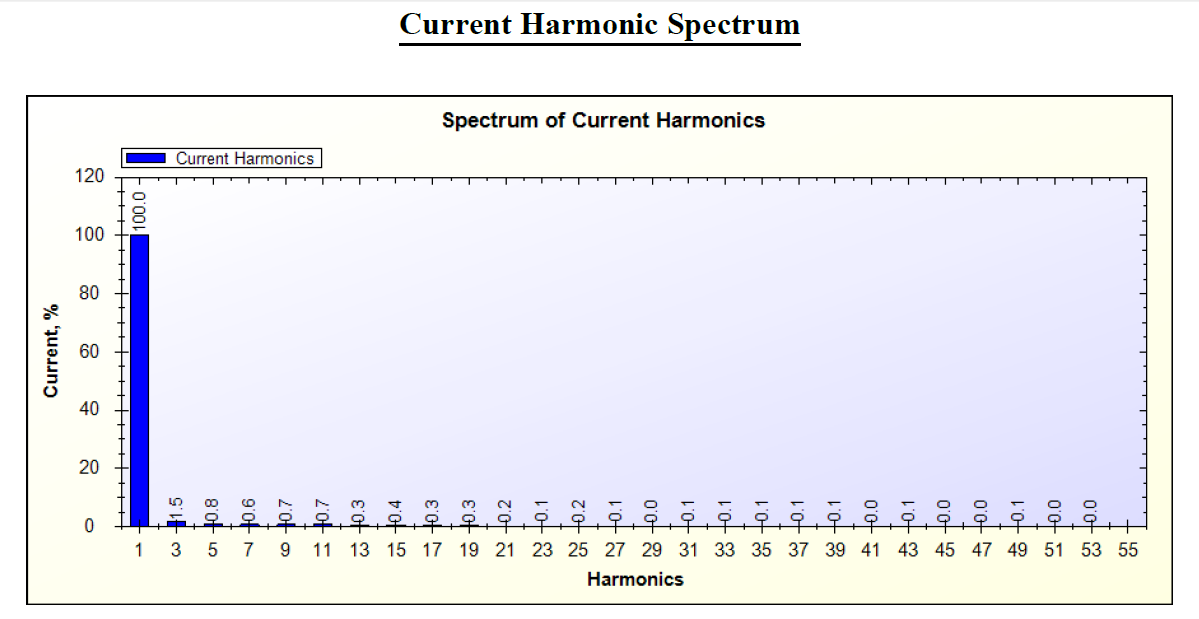Above is the Amps(Current) harmonics spectrum . All values are given in % of Arms.

Harmonics report is also generated which contains the parameter valuews with Harmonics spectrum.